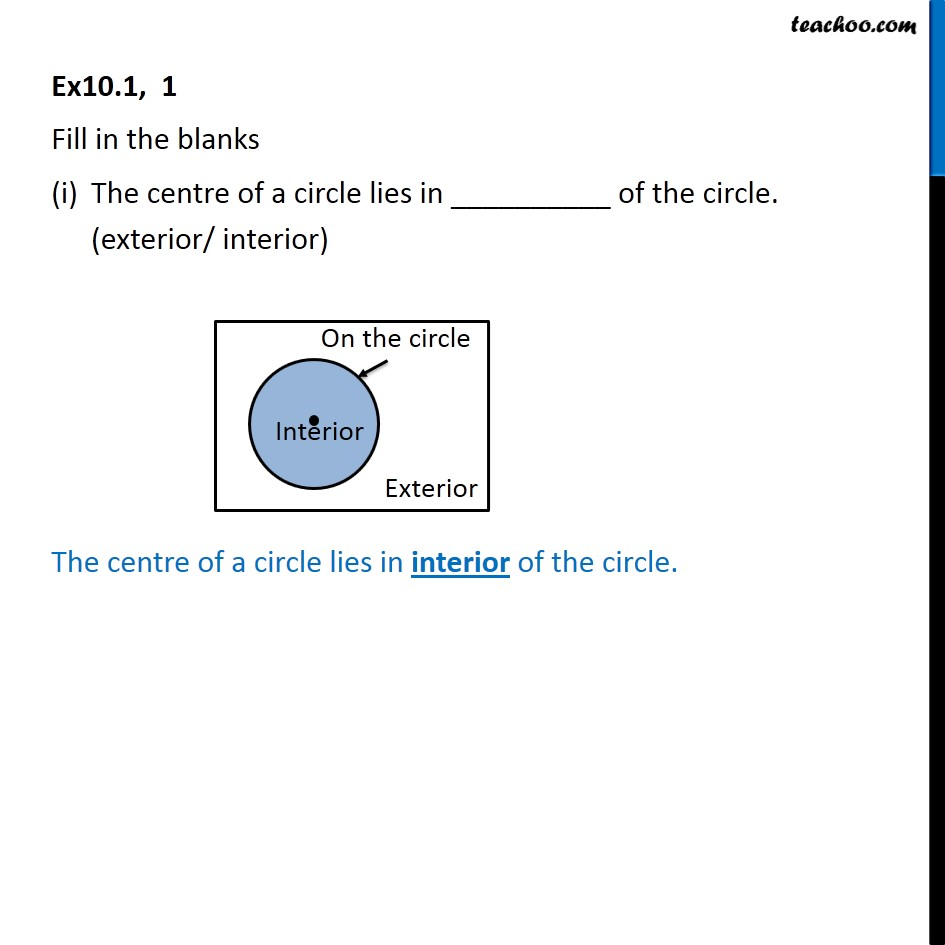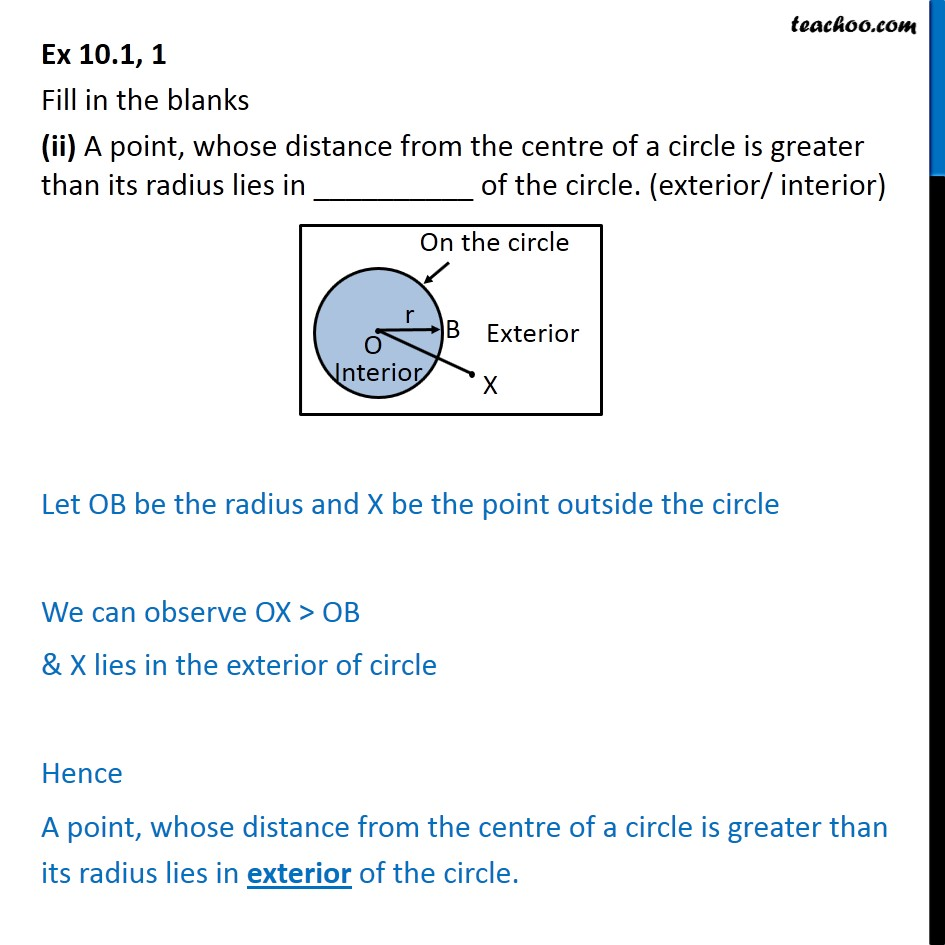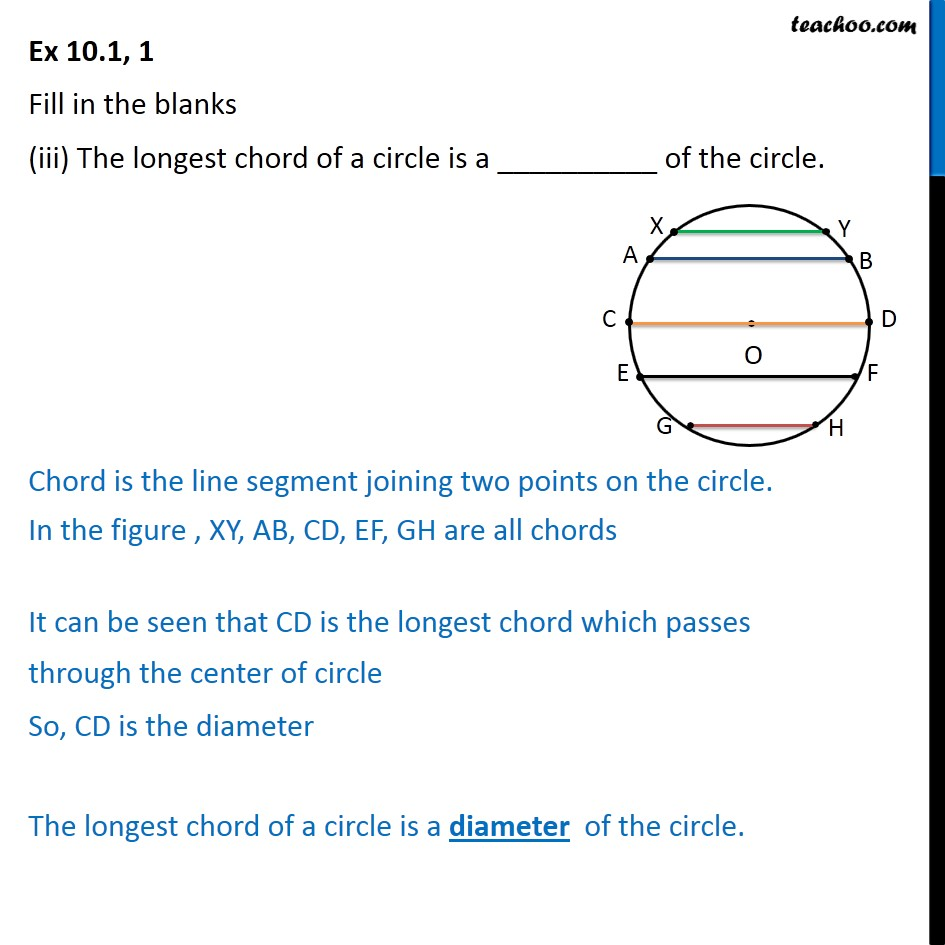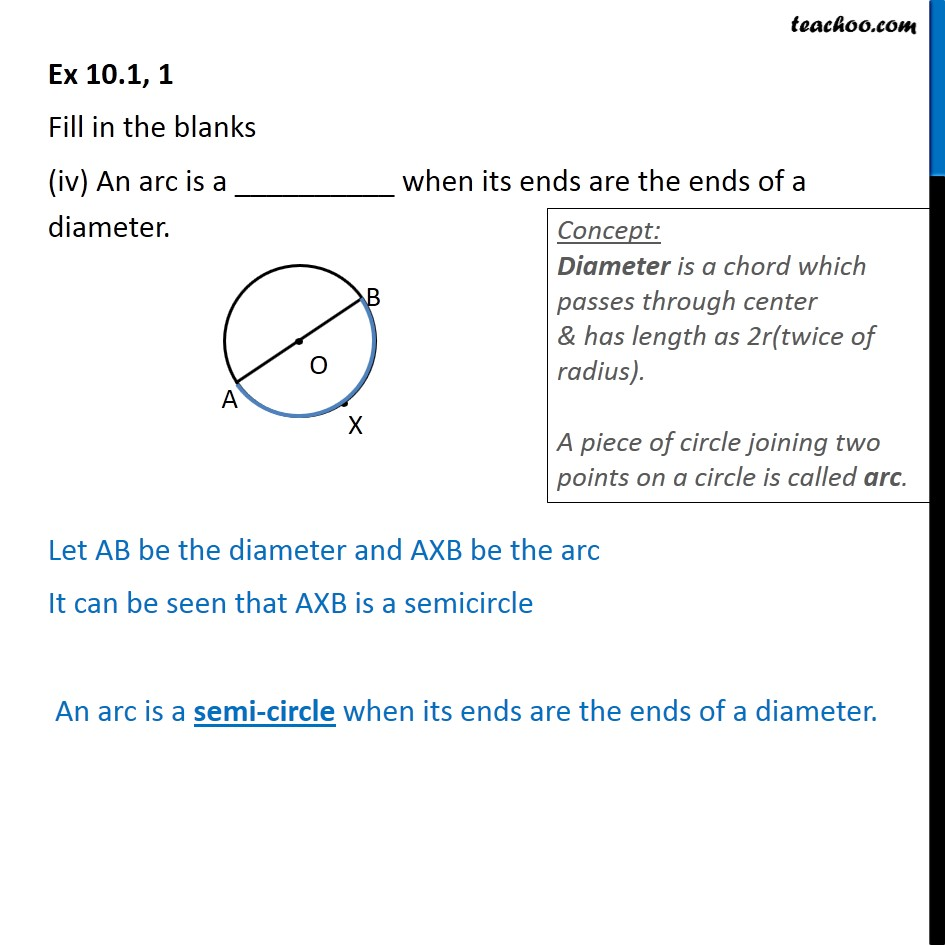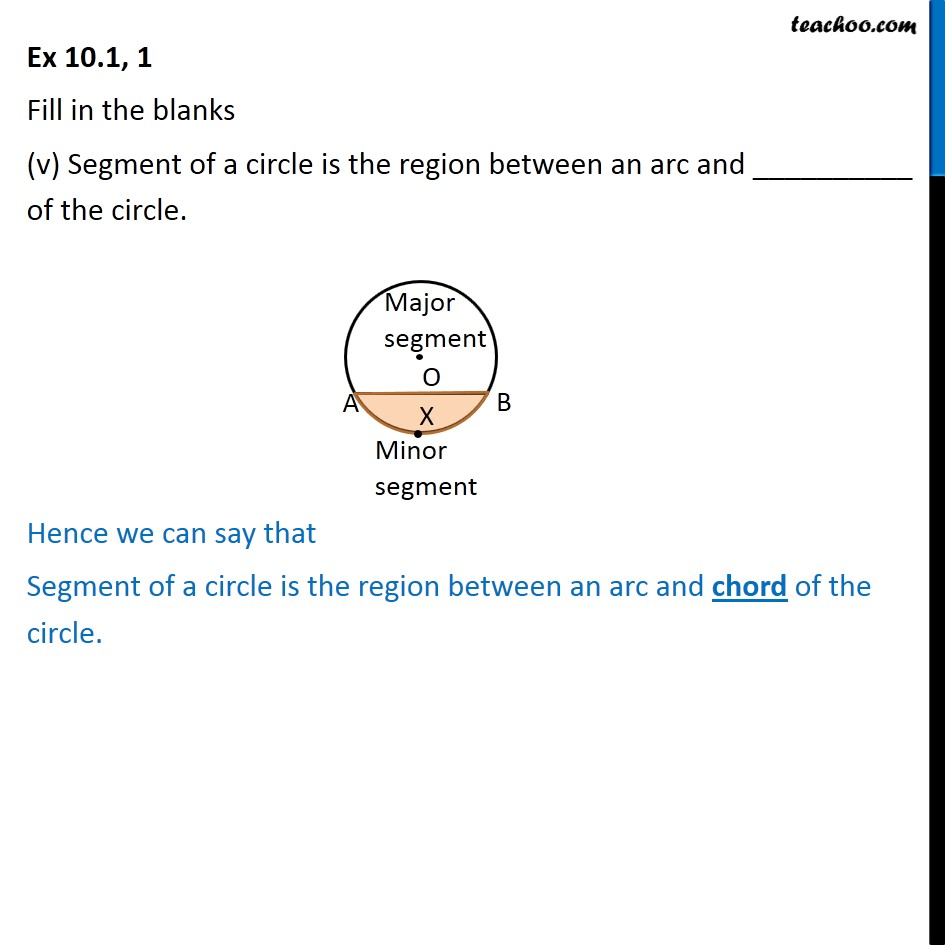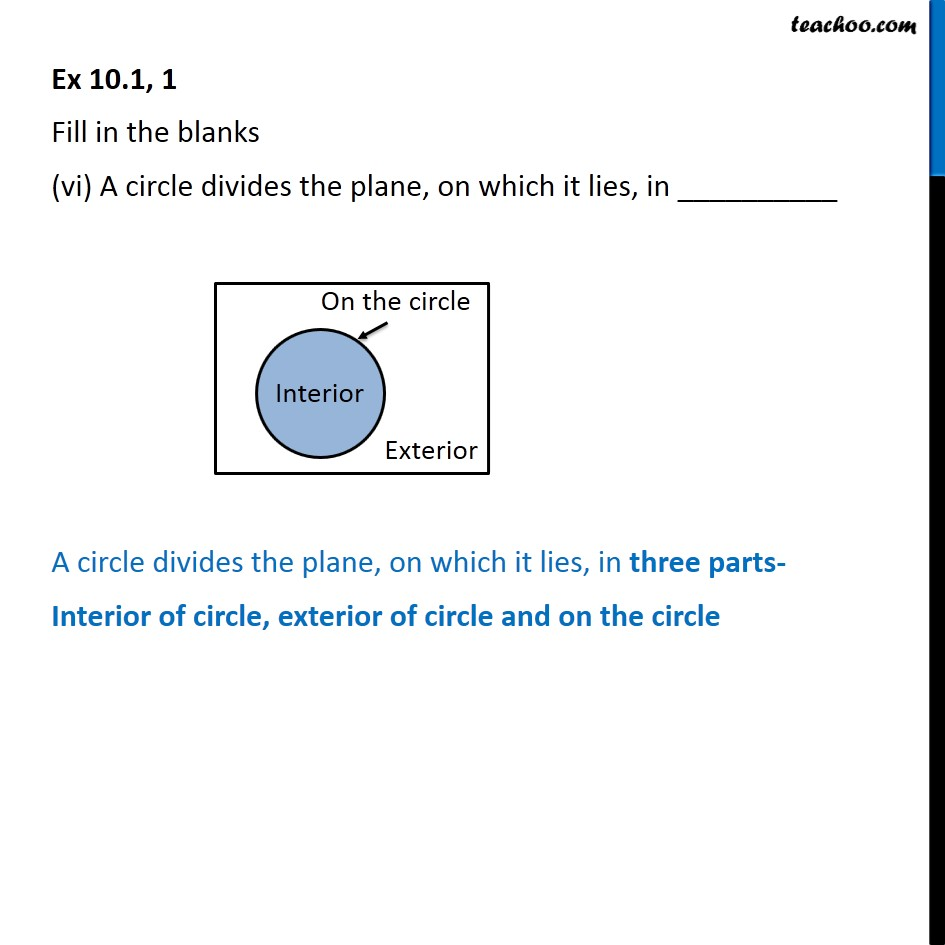1. Chapter 10 Class 9 Circles
2. Serial order wise
3. Ex 10.1

Transcript

Ex10.1, 1 Fill in the blanks The centre of a circle lies in __________ of the circle. (exterior/ interior) The centre of a circle lies in interior of the circle. Ex 10.1, 1 Fill in the blanks (ii) A point, whose distance from the centre of a circle is greater than its radius lies in __________ of the circle. (exterior/ interior) Let OB be the radius and X be the point outside the circle We can observe OX > OB & X lies in the exterior of circle Hence A point, whose distance from the centre of a circle is greater than its radius lies in exterior of the circle. Ex 10.1, 1 Fill in the blanks (iii) The longest chord of a circle is a __________ of the circle. Chord is the line segment joining two points on the circle. In the figure , XY, AB, CD, EF, GH are all chords It can be seen that CD is the longest chord which passes through the center of circle So, CD is the diameter The longest chord of a circle is a diameter of the circle. Ex 10.1, 1 Fill in the blanks (iv) An arc is a __________ when its ends are the ends of a diameter. Let AB be the diameter and AXB be the arc It can be seen that AXB is a semicircle An arc is a semi-circle when its ends are the ends of a diameter. Ex 10.1, 1 Fill in the blanks (v) Segment of a circle is the region between an arc and __________ of the circle. Hence we can say that Segment of a circle is the region between an arc and chord of the circle. Ex 10.1, 1 Fill in the blanks (vi) A circle divides the plane, on which it lies, in __________ A circle divides the plane, on which it lies, in three parts- Interior of circle, exterior of circle and on the circle

Ex 10.1

Chapter 10 Class 9 Circles
Serial order wise

About the AuthorDavneet Singh
Davneet Singh is a graduate from Indian Institute of Technology, Kanpur. He has been teaching from the past 10 years. He provides courses for Maths and Science at Teachoo.